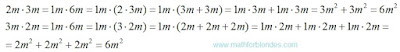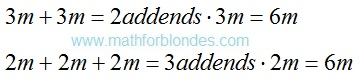## 8.14.2016

### Distinctions between multiplication and addition

Subject of occupations:
TRIGONOMETRIC FUNCTIONS IN A RECTANGLE
Subject of the previous lesson
Examples of multiplication

Lesson 10

Multiplication is a change of quality, that is change of units of measure. Contrary to the standard opinion, multiplication cannot be presented in the form of addition. When multiplication is substituted for addition, mathematical properties of multiplication are used. With units of measure substitution looks so:Multiplication substitution by addition

Algebraic expressions with use of letters show distinction between addition and multiplication:Multiplication in algebra

If at addition items have identical numerical values, then it is possible to apply mathematical model of multiplication for addition. At the same time we assume that units of measure at multiplication do not change:Addition replacement with multiplication

In mathematician possess separate properties of units of measure:

- number systems of numbers – it is impossible to addition the numbers presented in different number systems, a question of a possibility of multiplication of numbers in different number systems the author it was not studied;

- denominators of common fractions – it is impossible to addition fractions with different denominators, at multiplication of fractions denominators are multiplied;

- alphabetic references in algebraic expressions – it is impossible to addition numbers with different designations, at multiplication new designation of result turns out;

- legends of functions (for example, trigonometrical).

At the following lesson we will consider
Zero and infinity